Model Question: Compulsory Mathematics

Model Question: Compulsory Mathematics

MODEL QUESTION

Subject: Compulsory Mathematics

Full Marks: 100

Time: 3 hrs

*Candidates are required to answer in their own words as far as practicable.

*Credit will be given to originality, not rote learning.

Attempt ALL the questions.

Group 'A' [9×(2+2)=36]

1. a. Evaluate: $$\frac{2^x\times3-2^x}{2^{(x+2)}-2^{(x+1)}}$$

b. Simplify:  $$\sqrt{54}$$ - $$\sqrt{250}$$ + $$\sqrt{16}$$

2. a. Solve: $$\sqrt{9x^2 – 20}$$ = 3x – 2

b. Find three consecutive numbers such that 6 times the

smallest number is equal to double the sum of the other two.

3. a. If the mean of a grouped data  having fm = 300 is 18,

find the value of N.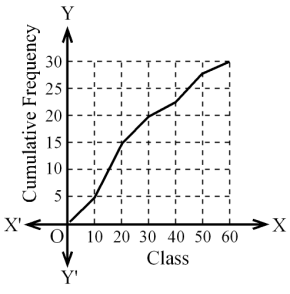b. Find the third quartile class from the given ogive.

4. a. A card is drawn from a well shuffled deck of 52 playing cards.

Find the probability that the card drawn is either a black card or a jack.

b. There are 5 black and 6 white balls of same shape and size in a box.

Two balls are drawn at random in succession without replacement from the box.

Show the probability of all possible outcomes in a tree diagram.

5. a. The area of the adjoining parallelogram ABCD is 20cm2.

If CD = 5cm and AD = 8cm, find the value of BCD.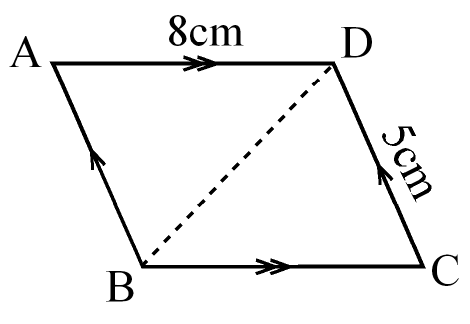b. The base of a right prism is an equilateral triangle with a perimeter of 12 cm.

The height of the prism is 7$$\sqrt{3}$$ cm. Calculate its volume.

6. a. The total surface area of a hemi-sphere is 462cm2. Find its diameter.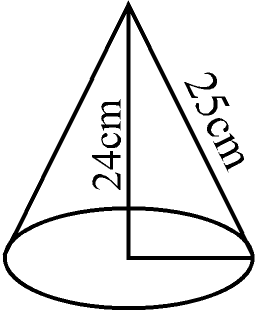b. Find the base area of the given cone.

7. a. The price of a shirt becomes Rs. 1485 including 10% VAT.

Calculate its marked price if a discount of 10% is given.

b. In how many years will the population of a town be 209475 from

190000 at the growth rate of 5% per annum?

8. a. In the given figure, PT // QR. Square PQRS and parallelogram SQRT

are on the same base QR. If the diagonal QS = 8cm, find the area of

parallelogram SQRT.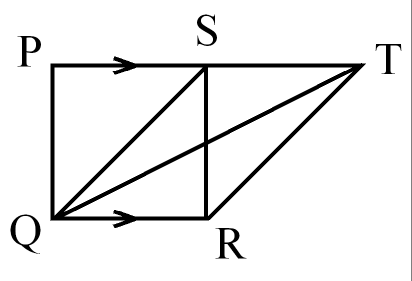b. In the given figure, O is the centre of the circle.

If BAC = 44º, find the value of OBC.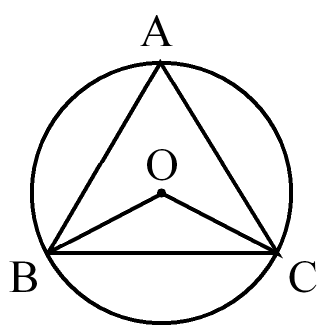9. a. Find the value of ‘x’ from the given figure.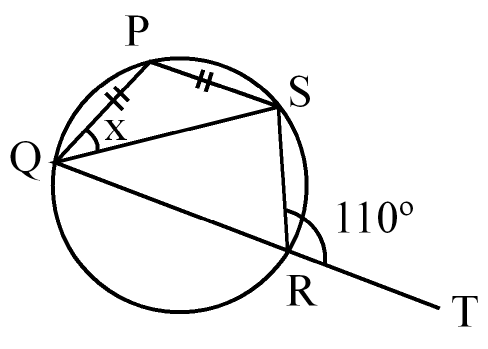b. In the given figure, O is the centre of the circle.

PM and PN are two tangents. If PS = 7cm and OS = 5cm,

find the length of PM and PN.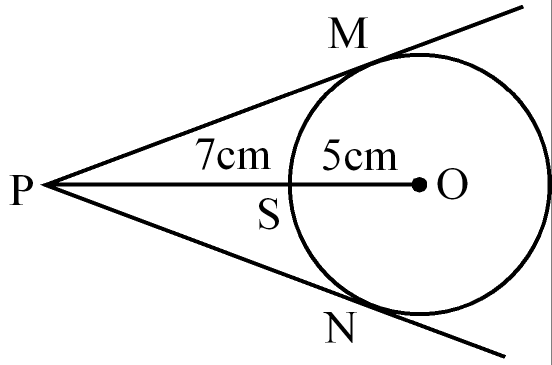Group 'B' [16×4 =64]

10. In an examination, 40% students passed in Mathematics,

45% passed in Science and 55% passed in English. 10% passed in

Mathematics and Science, 20% in Science and English

and 15 % in English and Mathematics.

a. Find the percentage of students who passed in all the three subjects.

b. Draw a Venn- diagram to illustrate the information.

11. Find the LCM: 2x3 + 16, x2 + 3x + 2 and x2 + 4x + 4.

12. Solve: 32x – 4 × 3x + 2 + 243 = 0

13. Simplify: $$\frac{1}{x-a}$$ - $$\frac{2}{2x+a}$$ + $$\frac{1}{x+a}$$ - $$\frac{2}{2x-a}$$

14. A fast bus takes 2 hours less than a slow bus for a journey of 240km.

If the speed of the slow bus is 10 km/hr less than the speed of the fast bus,

find the speed of both the buses.

15. If the mean of the following data is 41.5, find the missing frequency a.

 Class 10-20 20-30 30-40 40-50 50-60 60-70 Frequency (f) 3 4 a 15 3 5

16. A tree was broken due to the storm and the broken part bends

so that the top of the tree touches the ground making an angle of 30°

with the ground at a distance of 24 metres from the foot of the tree.

Find the height of the tree before it was broken.

17. Calculate the total surface area of the given combined solid.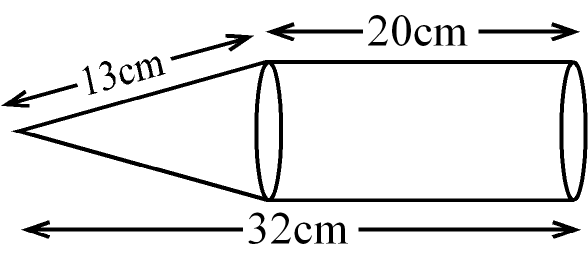18. The lateral surface area of a square-based pyramid is 720cm2

and its slant height is 30cm. Calculate the volume of the pyramid.

19. A, B and C can complete a piece of work in 30, 40 and 60 days

respectively. All of them started the work together but B left the

work after working for 8 days. In how many days A and C

complete the remaining work?

20. A computer is sold at Rs. 20700 after giving 10% discount

and adding 15% VAT. Calculate the VAT amount.

21. If a sum becomes Rs.13310 in three years and Rs.14641 in four

years,interest being compounded annually, calculate the sum and

the rate of interest.

22. QRT and parallelogram PQRS are on the same base QR and

between the same parallel lines QR // PS. Prove that area of

the parallelogram PQRS = 2 times the area of QRT.

23. In the given figure, PAR = QBS. Prove that PS // QR.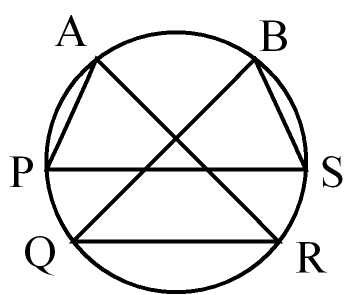24. Verify experimentally that the opposite angles of a cyclic

quadrilateral are supplementary. (Two figures are needed.

The radii of the circles should be more than 3cm.)